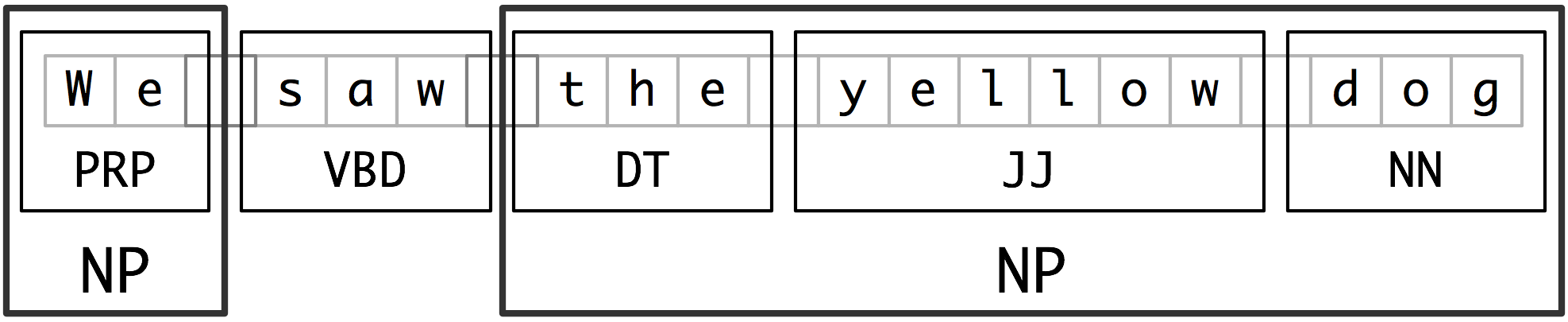# Lecture 2: Lexical association measures and hypothesis testing

• Lexical association
• Named entities: http://www.nltk.org/book/ch07.html
• Information extraction architecture
• raw text->sentence segmentation->takenization0<part of speech tagging->entity detection->relation detection
• chunking: segments and labels multi-token sequences as illustrated in 2.1.• Noun-phrase (NP) chunking
• tag patterns: describe sequences of tagged words
• Chunking with Regular Expressions
• Exploring Text Corpora
• Chinking: define a chink to be a sequence of tokens that is not included in a chunk
• Representing chunks: tags & trees
• Training Classifier-Based Chunkers
• Accurate methods for the statistics of surprise and coincidence
• An introduction to lexical association measures:
• We use the term lexical association to refer to the association between words.
• 3 types of association:
• collocational association: restricting combination of words into phrases
• semantic association: reflecting the semantic relationship between words
• d cross-language association: corresponding to potential translations of words between different languages
• Topics:
• Knowledge/ data->zipf
• Hypothesis testing
• Collations
• NER
• Knowledge/ data
• Rationalist:
• Empiricist: driven by observation
• Zipf’s law: the frequency of any word is inversely proportional to its rank in the frequency table.
•                                      Empiricist                          Rationalist
• core                              frequent
• periphery                     infrequent                     exception relation
• Example 1
• Alice like it. a book.
• Question: what does Alice like?
• Instead of using a string of word, use a tree structure to represent a sentence.
•              CP
•    spec           IP
• what         Alice like
• Example 2 (Rationalist)
• Which book did mary say that Bill thinks Alice like?
• He dropped a book about info theory.
• What did he drop a book about? (questionable) What did he write a book about? (Good)
• He dropped [a book about info theory].
• S                                   NP
• Example 3
•        SBarQ
• WhNP                             SQ
• WP           VR           NP             VBR                   NP
•  what         odes     mary              read                  T*-1
• Balance between rationalist and empiricist
• Accuracy vs. coverage
• Data-driven algorithms less concerned with over generation
• Semi-supervised learning
• How to relate the algorithm to the wat we learned.
• 80-20 rule
• Remove the low-frequency word and focus on the frequent words for the structure.
• Hypothesis testing (Chi^2 test)
• P(H|E) H: hypothesis, E: evidence
• P(q = 0.7 | 76 success, 24 failures)
• pmf:
• R = P(H1|E) / P(H2|E)
• Recipe for statistical hypothesis
• Step 1: H0: null hypothesis (what we think is not true)
• Example: coin flip: coin has only one side.
• Step 2: Test statistic: number we compute based on evidence collection
• Step 3: Identify test statistics distribution: if H0 were true.
• Step 4: Pick a threshold to convince that the H0 is not true.
• Step 5: Do an experiment and calculate the test statistic.
• Step 6: If it sufficiently improbable assuming H0 is true, should reject H0.
• H0: q = 0.05, H1: q != 0.05
• Example:
• H0: P(H) = 0.05
• R~Binomial(n, p)
• Likelihood func: b(r,n,p) = C(n r) p^r (1-p)^(n-r)